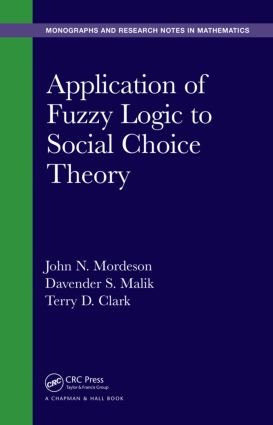# Application of Fuzzy Logic to Social Choice Theory

## 1st Edition

Chapman and Hall/CRC

352 pages

##### Purchasing Options:\$ = USD
Hardback: 9781482250985
pub: 2015-03-03
SAVE ~\$26.00
\$130.00
\$104.00
x
eBook (VitalSource) : 9780429157905
pub: 2015-03-03
from \$28.98

FREE Standard Shipping!

### Description

Fuzzy social choice theory is useful for modeling the uncertainty and imprecision prevalent in social life yet it has been scarcely applied and studied in the social sciences. Filling this gap, Application of Fuzzy Logic to Social Choice Theory provides a comprehensive study of fuzzy social choice theory.

The book explains the concept of a fuzzy maximal subset of a set of alternatives, fuzzy choice functions, the factorization of a fuzzy preference relation into the "union" (conorm) of a strict fuzzy relation and an indifference operator, fuzzy non-Arrowian results, fuzzy versions of Arrow’s theorem, and Black’s median voter theorem for fuzzy preferences. It examines how unambiguous and exact choices are generated by fuzzy preferences and whether exact choices induced by fuzzy preferences satisfy certain plausible rationality relations. The authors also extend known Arrowian results involving fuzzy set theory to results involving intuitionistic fuzzy sets as well as the Gibbard–Satterthwaite theorem to the case of fuzzy weak preference relations. The final chapter discusses Georgescu’s degree of similarity of two fuzzy choice functions.

Fuzzy Maximal Subsets

Fuzzy Set Theory

Fuzzy Maximal Sets

Fuzzy Choice Functions

Basic Properties

Consistency Conditions

M-Rationality and G-Rationality

Full Rationality of Fuzzy Choice Functions on Base Domain

Quasi-Transitive Rationality of Fuzzy Choice Functions

Full Rationality and Congruence Axioms of Fuzzy Choice Functions

Factorization of Fuzzy Preference Relations

Basic Definitions and Results

Quasi-Subtraction

Factorizations

Intuitionistic Fuzzy Relations

Intuitionistic Fuzzy Preference Relations and Their Factorization

Fuzzy Non-Arrow Results

Nondictatorial Fuzzy Aggregation Rules

Auxiliary Functions

Arrow’s Theorem and Max-Star Transitivity

Fuzzy Arrow’s Theorem

Dictatorial Fuzzy Preference Aggregation Rules

Representation Rules, Veto Players, Oligarchies, and Collegiums

Decisive Sets, Filters, and Fuzzy Arrow’s Theorem

Fuzzy Preferences and Social Choice

Fuzzy Preferences and Arrow-Type Problems

The Structure of Fuzzy Preferences: Social Choice Implications

Single Peaked Fuzzy Preferences: Black’s Median Voter Theorem

Fuzzy Aggregation Preference Rules

Fuzzy Voting Rules

Single-Peaked Fuzzy Profiles

The Core in Fuzzy Spatial Models

Rationality

Fuzzy Preference and Preference-Based Choice Functions

Fuzzy Choice Functions, Revealed Preference and Rationality

Arrow-Type Results under Intuitionistic Fuzzy Preferences

Fuzzy Preference Profiles and Fuzzy Aggregation Rules

IIA3 and Nondictatorial Fuzzy Aggregation Rules

IIA2, IIA4, and Fuzzy Arrow’s Theorem

Representation Rules, Veto Players, and Oligarchies

Fuzzy Preference and Arrowian Results

Intuitionistic Arrow’s Theorem and Gibbard’s Oligarchy Theorem

Manipulability of Fuzzy Social Choice Functions

Preliminaries

Fuzzy Social Choice Functions

Impossibility Results

Non-Manipulable Partitioning

Application

Similarity of Fuzzy Choice Functions

Fuzzy Choice Functions

Similarity of Fuzzy Choice Functions

Arrow Index of a Fuzzy Choice Function

Index

Exercises and References appear at the end of each chapter.

### About the Authors

Dr. John N. Mordeson is a professor of mathematics, the John N. Mordeson Endowed Chair in Mathematics, and the director of the Center for Mathematics of Uncertainty at Creighton University. Dr. Mordeson has published 15 books and nearly 200 journal articles. He is an editorial board member of numerous journals and the current president of the Society for Mathematics of Uncertainty. His research interests include coding theory, fuzzy automata theory, fuzzy information retrieval, and fuzzy cluster analysis.

Dr. Davender S. Malik is a professor of mathematics at Creighton University. His research interests include algebra, fuzzy automata theory, and fuzzy logic. Dr. Malik has published 17 books and more than 50 papers.

Dr. Terry D. Clark is a professor of political science and the director of the graduate program in international relations at Creighton University. Dr. Clark has published four books and numerous journal articles. His research interests include fuzzy math spatial modeling, comparative politics, international relations, social network analysis, and intelligence analysis.

### Subject Categories

##### BISAC Subject Codes/Headings:
MAT003000
MATHEMATICS / Applied
MAT004000
MATHEMATICS / Arithmetic
MAT028000
MATHEMATICS / Set Theory